Build a deep neural network with ReLUs and Softmax.
Building a Deep Neural Network

## Deep Neural Networks: Introduction¶

Linear Model Complexity

• If we have N inputs and K outputs, we would have:
• (N+1)K parameters
•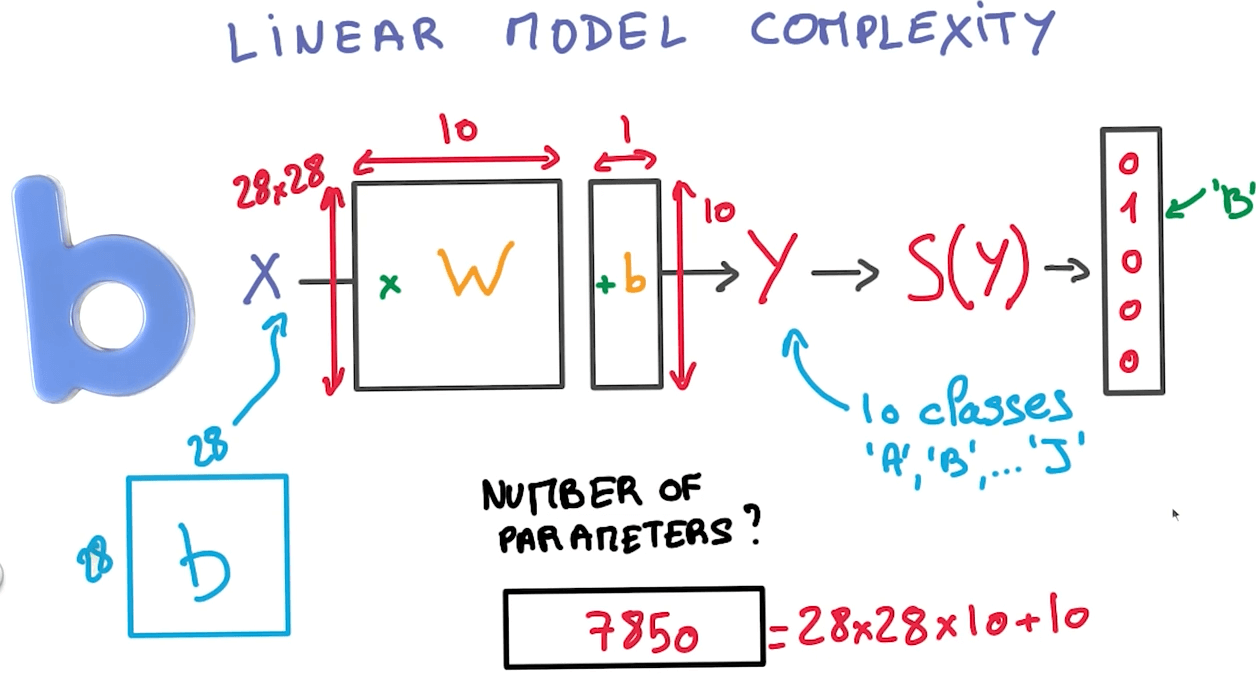• Limitation
• $y = x_1 + x_2$ can be represented well
• $y = x_1 * x_2$ cannot be represented well
• Benefits
• Derivatives are constants

Rectified Linear Units (ReLUs)

• This is a non-linear function.
•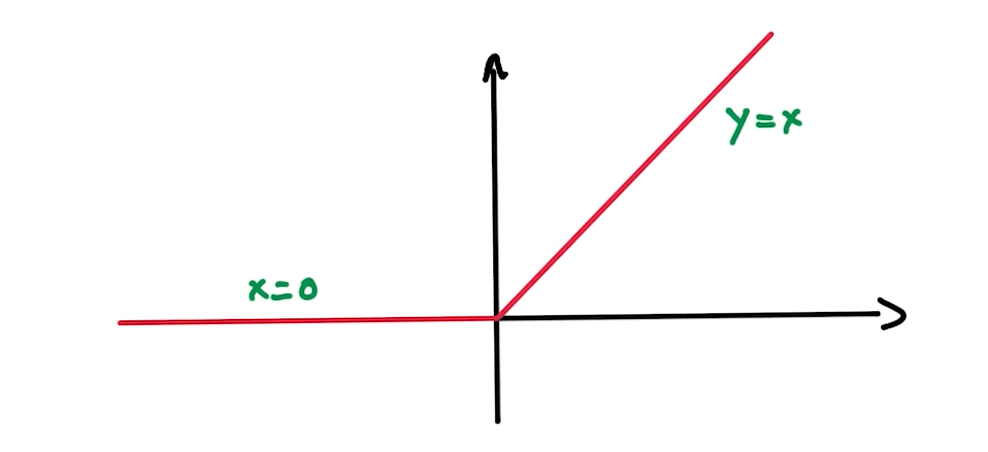• Derivatives are nicely represented too.
•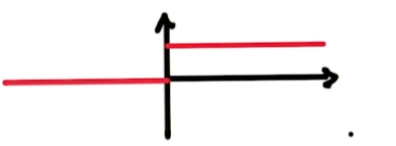Network of ReLUs: Neural Network

• We can do a logistic classifier and insert a ReLU to make a non-linear model.
•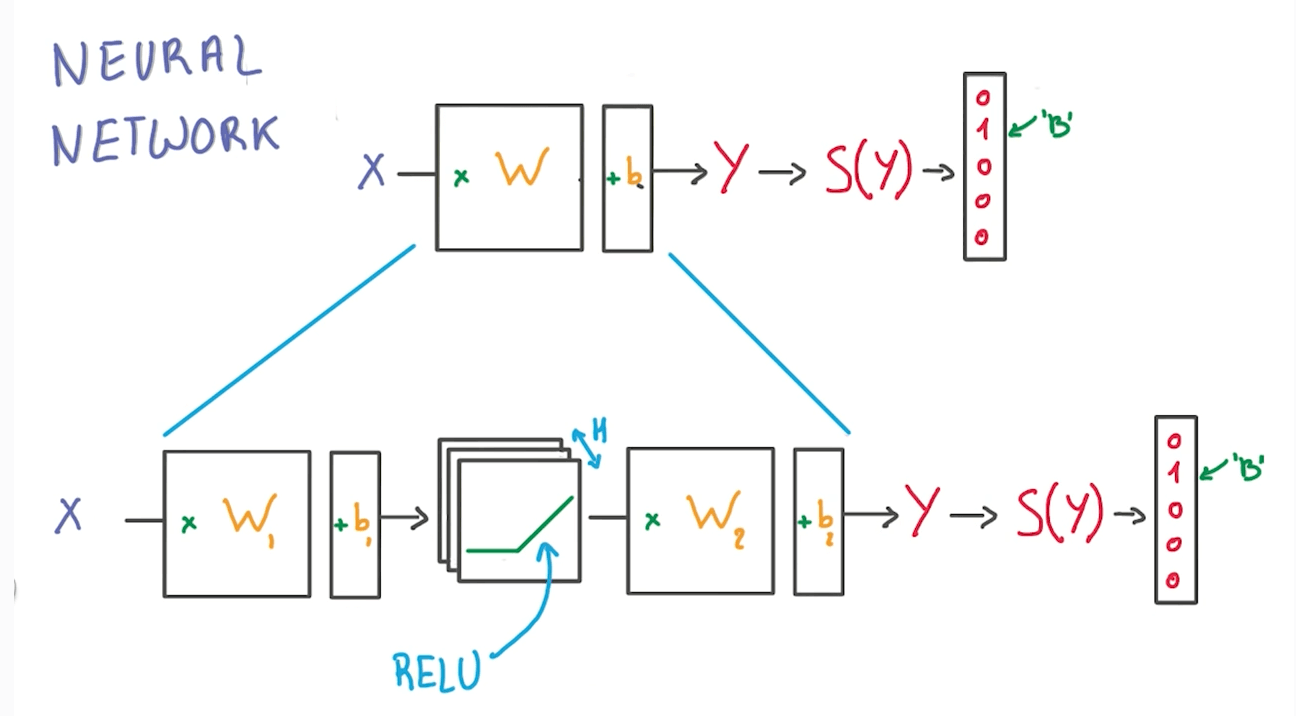• H: number of RELU units

2-Layer Neural Network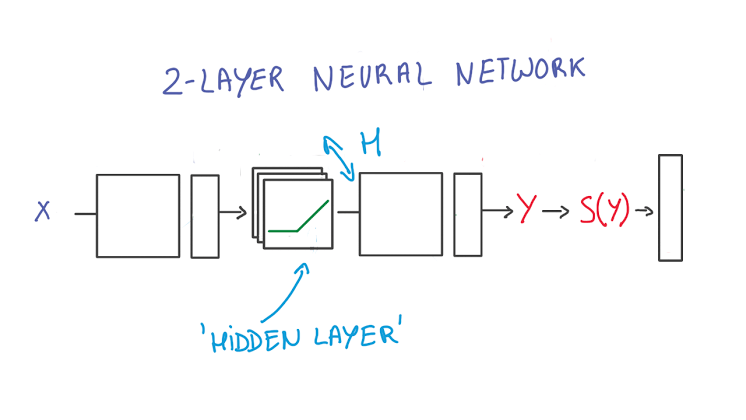1. The first layer effectively consists of the set of weights and biases applied to X and passed through ReLUs. The output of this layer is fed to the next one, but is not observable outside the network, hence it is known as a hidden layer.
2. The second layer consists of the weights and biases applied to these intermediate outputs, followed by the softmax function to generate probabilities.
• A softmax regression has two steps: first we add up the evidence of our input being in certain classes, and then we convert that evidence into probabilities.

Stacking Simple Operations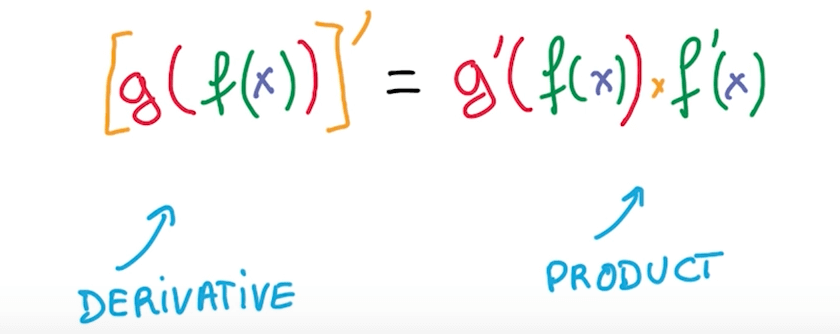• We can compute derivative of function by taking product of derivatives of components.

Backpropagation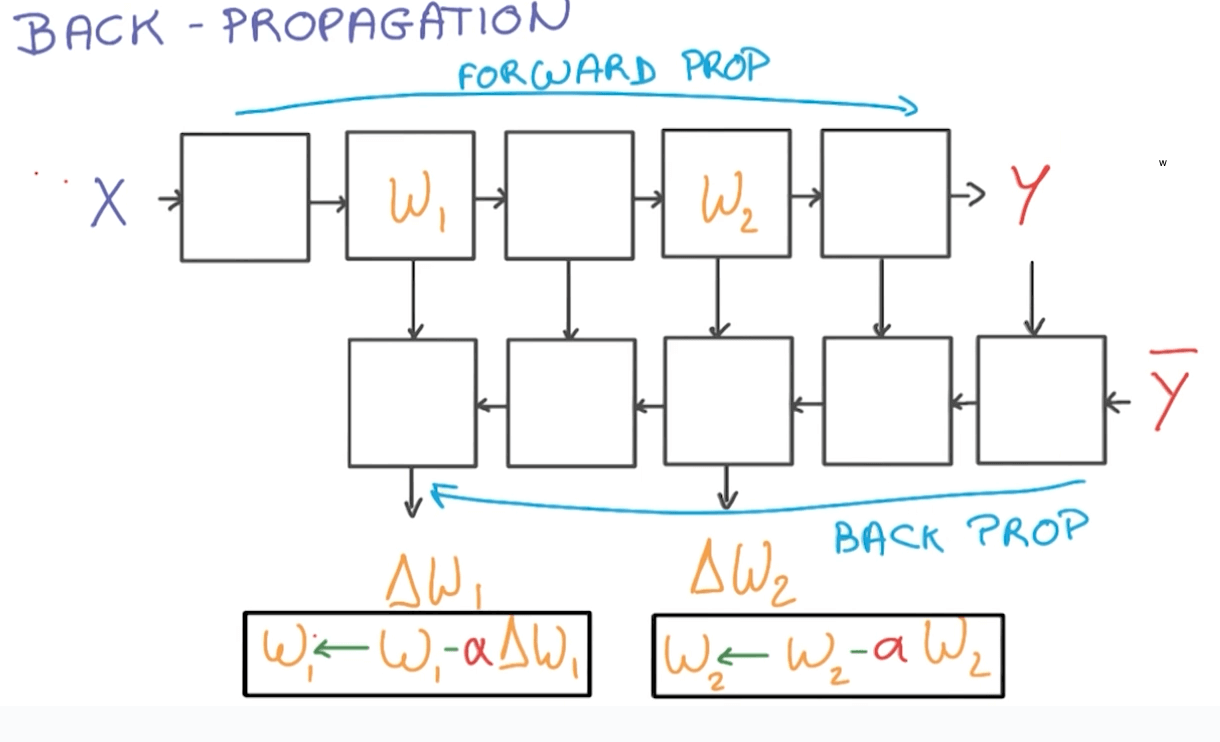• Forward-propagation
• You will have data X flowing through your NN to produce Y.
• Back-propagation
• Your labelled data Y flows backward to calculate "errors" of our calculations.
• You will be calculating the gradients ("errors"), multiply it by a learning rate, and use it to update our weights.
• We will be doing this many times.

Go Deeper

• It is better go deeper than increasing the size of the hidden layers (by adding more nodes)
• It gets hard to train.
• We should go deeper by adding more hidden layers.
• You would reap parameter efficiencies.
• However you need large datasets.
• Also, deep models can capture certain structures well such as the following.
•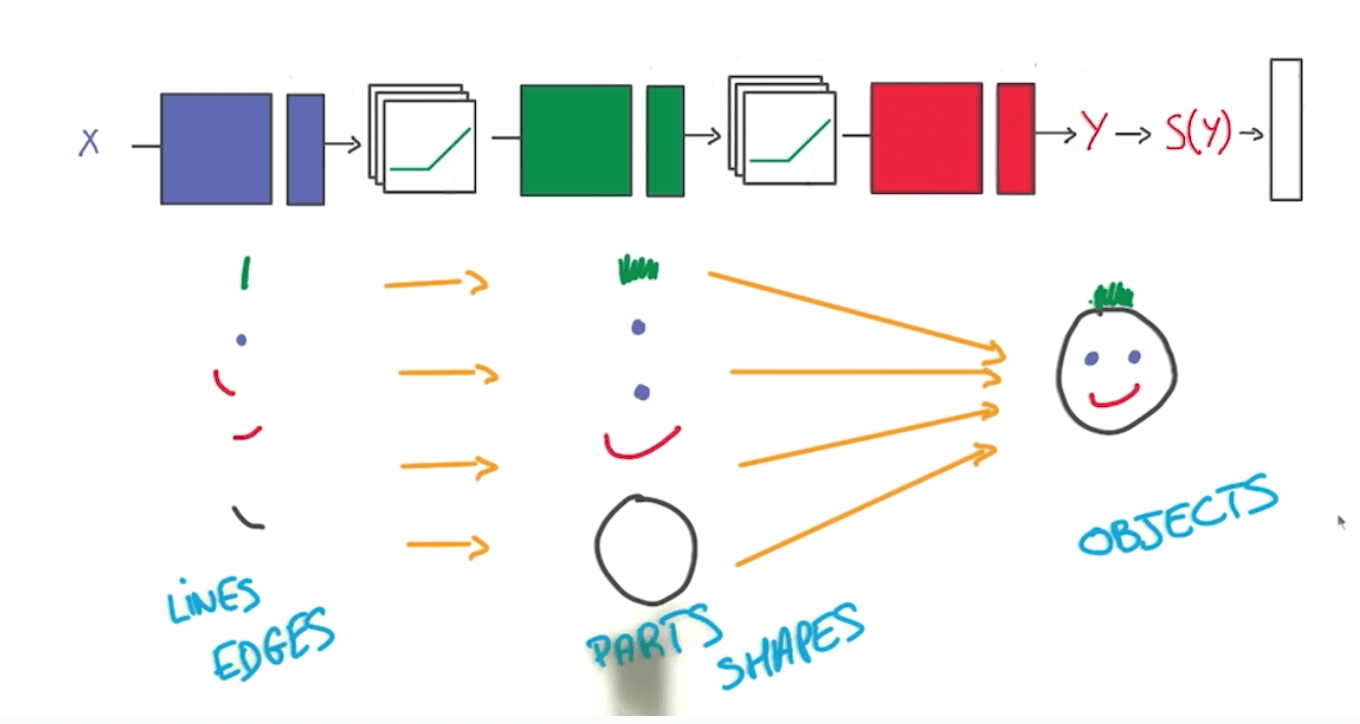Regularization

• We normally train networks that are bigger than our data.
• Then we try to prevent overfitting with 2 methods.
• Early termination
•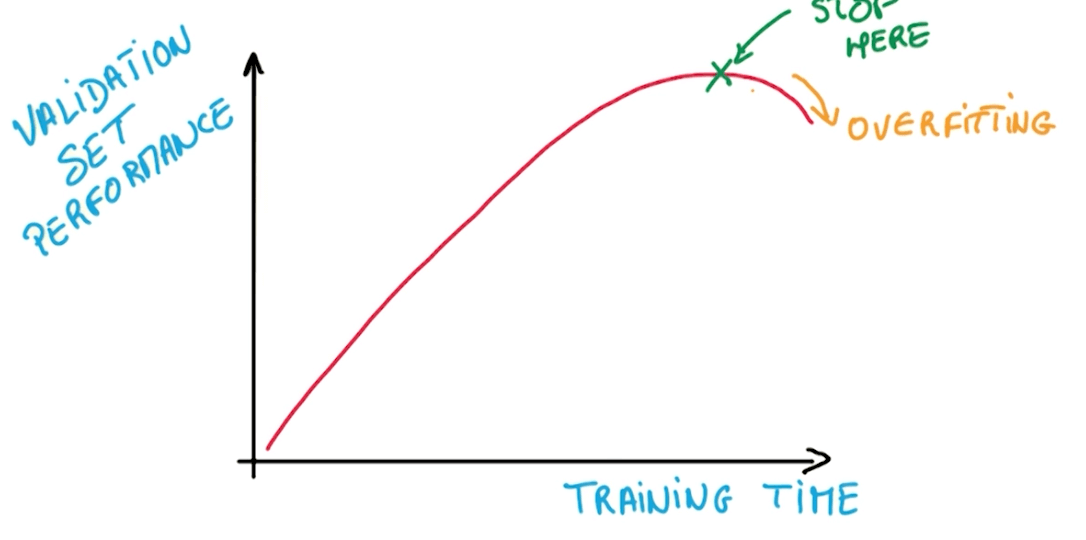• Regularization
• Applying artificial constraints.
• Implicitly reduce number of free parameters while enabling us to optimize.
• L2 Regularization
• We add another term to the loss that penalizes large weights.
• This is simple because we just add to our loss.
•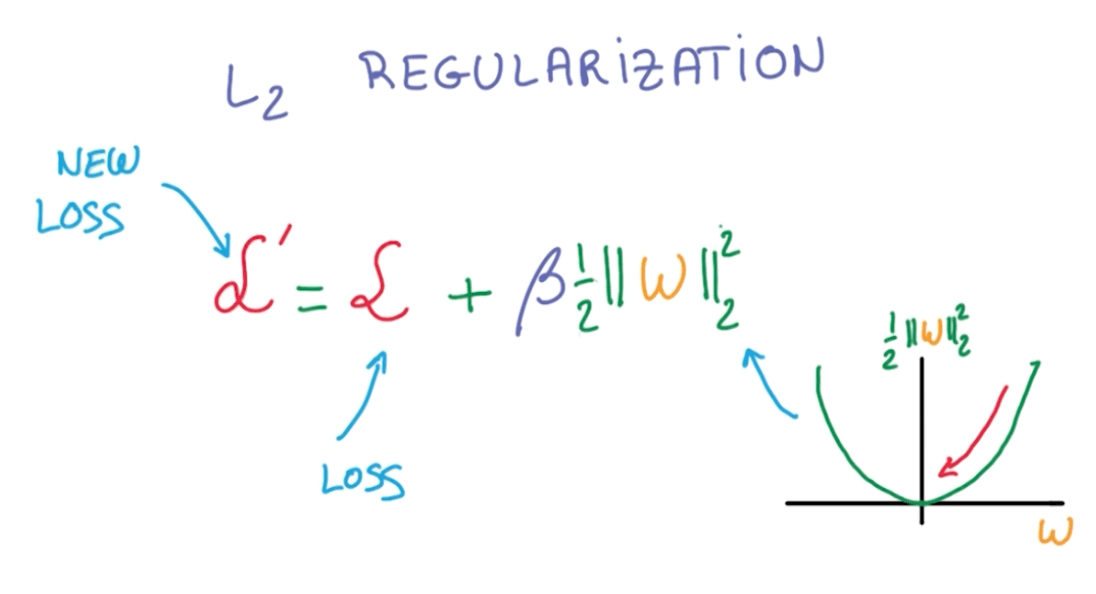L2 Regularization's Derivative

• The norm of w is the sum of squares of the elements in the vector.
• The equation:
•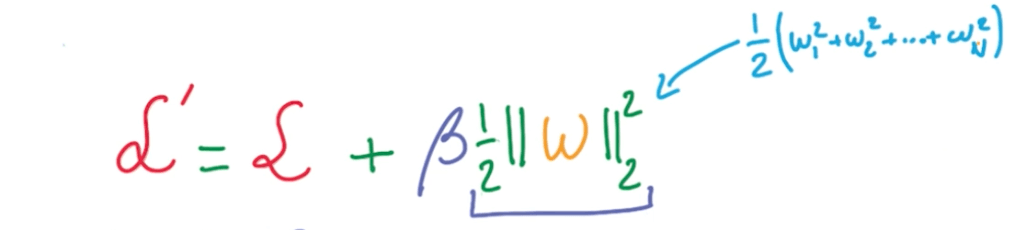• The derivative:
• $(\frac {1}{2} w^2)' = w$

L2 Regularizatin: Dropout

• Your input goes through an activation function.
• During the activation function, we randomly take half of the data and set to 0.
• We do this multiple times.
•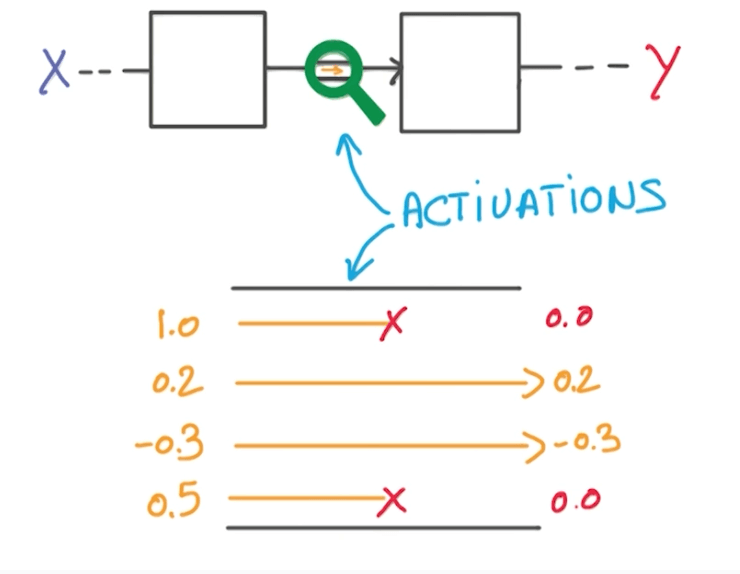• We are forced to learn redundant information.
• It's like a game of whack-a-mole.
• There's always one or more that represents the same thing.
•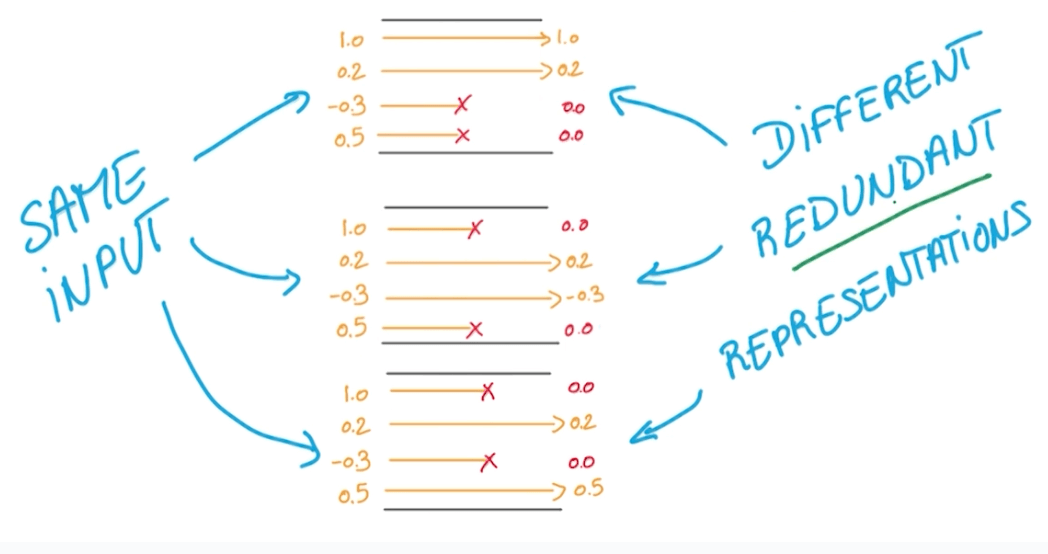• Benefits
• It prevents overfitting.
• It makes network act like it's taking a consensus of an ensemble of networks.

Dropout during Evaluation

• We would take the expectation of our training y's.
•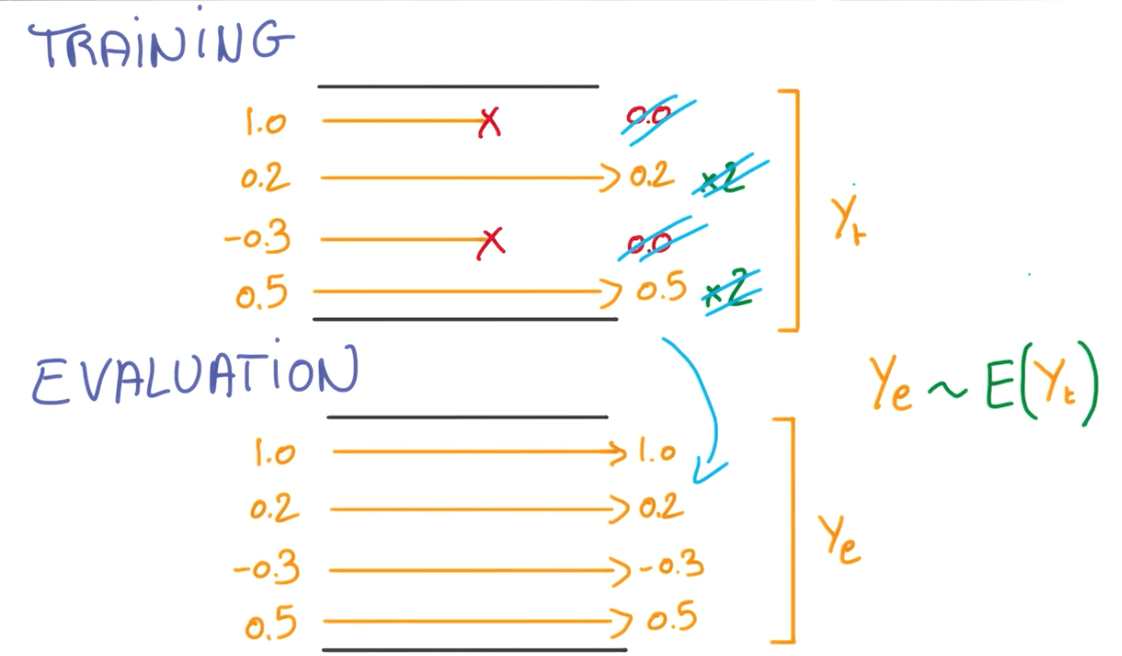Tags: